GRE Subject Test: Math : Substitution

Example Questions

Example Question #1 : Integrals

Integrate: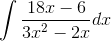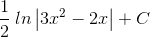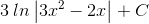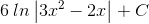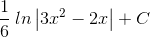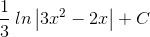Explanation:

This problem requires U-Substitution.  Let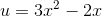and find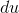.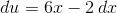Notice that the numerator inhas common factor of 2, 3, or 6.  The numerator can be factored so that theterm can be a substitute. Factor the numerator using 3 as the common factor.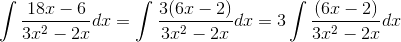Substitute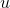andterms, integrate, and resubstitute theterm.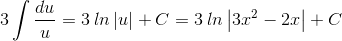Example Question #31 : Derivatives & Integrals

Evaluate the following integral: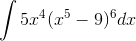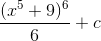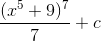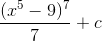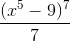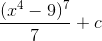Explanation:

To calculate this integral, we could expand that whole binomial, but it would be very time consuming and a bit of a pain. Instead, let's use u substitution:

Given this:We can say that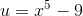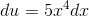Then, plug it back into our original expression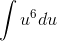Evaluate this integral to get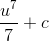Then, replace u with what we substituted it for to get our final answer. Note because this is an indefinite integral, we need a plus c in it.Example Question #3 : Integrals

Integrate the following using substitution.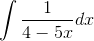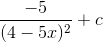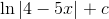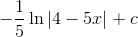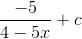Explanation:Using substitution, we set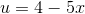which means its derivative is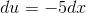.

Substitutingfor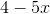, and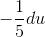for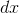we have: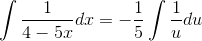Now we just integrate: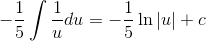Finally, we remove our substitutionto arrive at an expression with our original variable:Example Question #1 : Integrals

Evaluate the following integral:Explanation:

To calculate this integral, we could expand that whole binomial, but it would be very time consuming and a bit of a pain. Instead, let's use u substitution:

Given this:We can say thatThen, plug it back into our original expressionEvaluate this integral to getThen, replace u with what we substituted it for to get our final answer. Note because this is an indefinite integral, we need a plus c in it.All GRE Subject Test: Math Resources